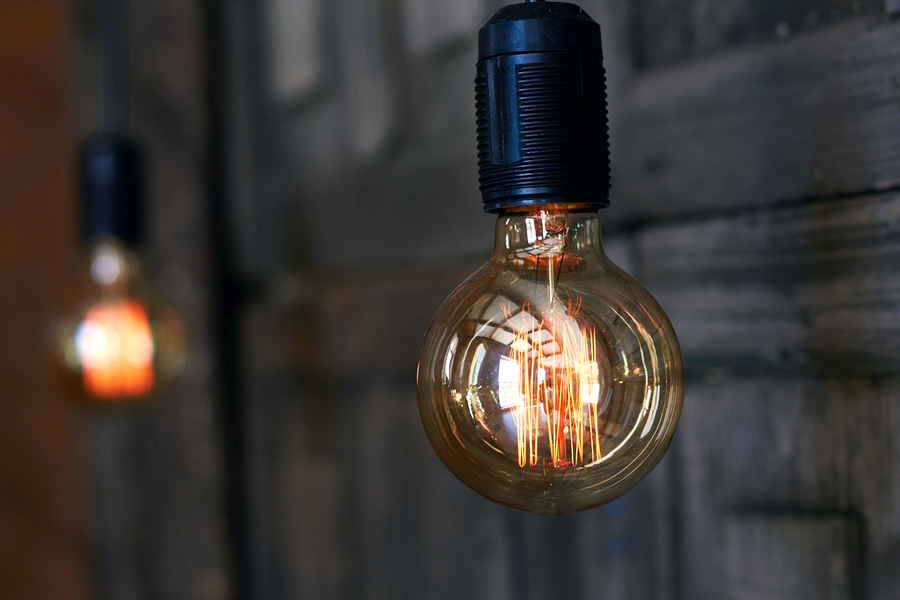Blog / Watt's a kWh? Getting to grips with energy units

# Watt's a kWh? Getting to grips with energy units

21st September 2018

Despite playing such an important role in powering our day-to-day lives, energy can be difficult to understand.

But for those who want a clearer understanding of their bill, or even those who are just curious about how energy use is measured, it’s interesting to take a dive into the world of amps, volts, watts and watt-ever else you can think of.## How electricity and gas is measured

There are different units at play when it comes to working out your energy. Electricity and gas are both measured in watts (w), kilowatts (kw), and kilowatt-hours (kWh).

Watts and kilowatts (one thousand watts) are measures of electricity volume: how much electricity it takes to power something.

For customers, kWh will be the most familiar. This is how your energy is measured as it passes your meter. It’s a measure of energy use over a length of time.

Say, for example, you have a 50-watt TV. It would take 20 hours to rack up 1 kWh of energy consumption. If you have a 100-watt fridge, it would take 10 hours to reach 1 kWh of consumption.

In effect, the amount of power you draw from the grid is measured in watts and kilowatts, and kilowatt-hours (kWh) refer to the amount of power drawn for a length of time.

Then, there are amps and ohms. These don’t play a part in your electricity bill, but are related to the current theme (get it?) Amps measure current, which is the flow of electricity, like measuring the flow of water in a river. Ohms measures resistance, which slows down the flow of current (and helps to stop your lightbulb from blowing because of too much power all at once).

Once you’ve wrapped your head around the energy units, you can multiply them by the energy requirements of a piece of equipment to work out how much that device is costing you.

There is a simple equation which allows you to work out how much your energy consumption is costing you. That equation is:

#### Power (kw) x Time (hours) = Energy Transferred (kWh)

Using these formulae, we can work out how much it costs to power a device.

To do so, you need to know how much power a device draws when plugged in. The average smartphone, for example, uses a 5w (or 0.005kw) charger. It takes three hours to charge fully. So, 0.005 (kw) x 3 (hrs) = 0.015 (kWh).

You then multiply your kWh figure by your unit rate to get the daily cost. With the UK average of 15p per kWh, the equation is 0.015 (kWh) x 0.15(p) = 0.00225p per charge. If you take 300 charges per year as an estimate, then the average smartphone costs… around 67p per year to charge.

If you don’t want to do the maths manually, you can use this free calculator to work out how much your appliances cost you to run.

For more help understanding your bill, see here.

## How smart meters take the stress out of energy

Smart meters are making life easier for energy users. With no need for manual meter reading, energy users get a simpler understanding, and better control, of their energy. You’ll be able to see exactly how many kwh you’re using each month.

What’s more, smart meters will help to create a cleaner and greener energy network for everyone by helping to facilitate a shift towards a smart grid which will better integrate renewable energy sources.

Smart meters are available to Opus Energy customers free of charge. If you’re an Opus Energy customer and you’re interested in getting a smart meter fitted at your premises, let us know here and we’ll take care of it.